# Machine Learning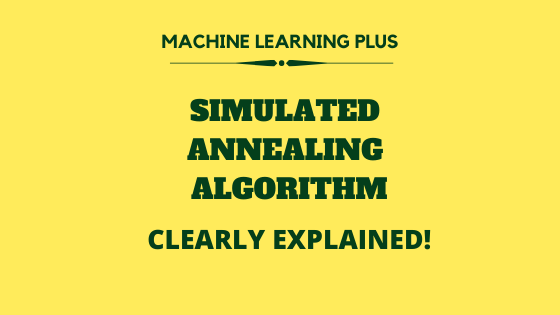## Simulated Annealing Algorithm Explained from Scratch (Python)

Simulated annealing algorithm is a global search optimization algorithm that is inspired by the annealing technique in metallurgy. In this one, Let’s understand the exact algorithm behind simulated annealing and then implement it in Python from scratch. First, What is Annealing? In simple terms, ‘Annealing’ is a technique, where a metal is heated to a …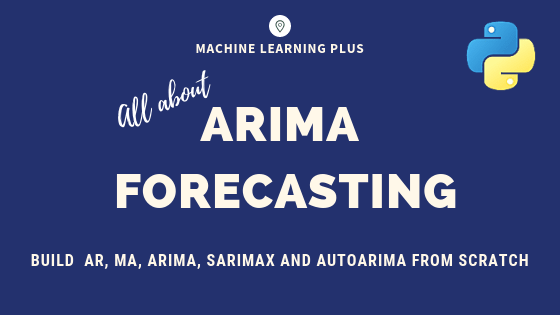## ARIMA Model – Complete Guide to Time Series Forecasting in Python

Using ARIMA model, you can forecast a time series using the series past values. In this post, we build an optimal ARIMA model from scratch and extend it to Seasonal ARIMA (SARIMA) and SARIMAX models. You will also see how to build autoarima models in python Master complete Time Series Concepts and Implementation with my …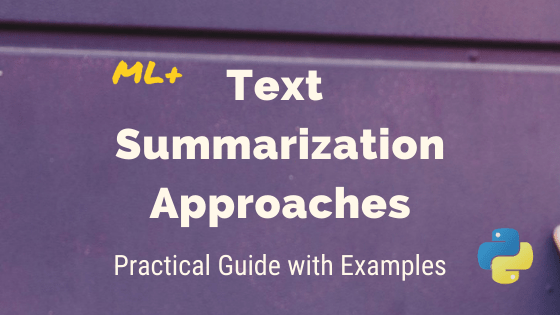## Text Summarization Approaches for NLP – Practical Guide with Generative Examples

Text summarization in NLP is the process of summarizing the information in large texts for quicker consumption. In this article, I will walk you through the traditional extractive as well as the advanced generative methods to implement Text Summarization in Python. Become a high paid data scientist with my structured Machine Learning Career Path. Includes …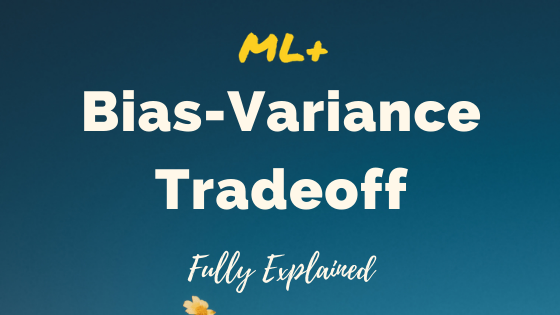## Bias Variance Tradeoff – Clearly Explained

Bias Variance Tradeoff is a design consideration when training the machine learning model. Certain algorithms inherently have a high bias and low variance and vice-versa. In this one, the concept of bias-variance tradeoff is clearly explained so you make an informed decision when training your ML models Bias Variance Tradeoff – Clearly Explained. Photo by …## Logistic Regression in Julia – Practical Guide with Examples

Julia is a powerful programming language for Machine Learning and Logistic regression is one of the most popular predictive modeling algorithms, used for binary classification. In this one, you will see the full work flow of how to implement churn modeling using Logistic regression in Julia. Logistic Regression with Julia. Photo by Sergio. This is …

## Confidence Interval – Fully Explained

Confidence interval is a measure to quantify the uncertainty in an estimated statistic (like the mean) when the true population parameter is unknown. Training Custom Text Classification Model in spaCy. Photo by Jessica Wong. You will know 1. What is Confidence Interval? 2. Two types of Confidence Intervals problems 3. Difference between Population parameter vs …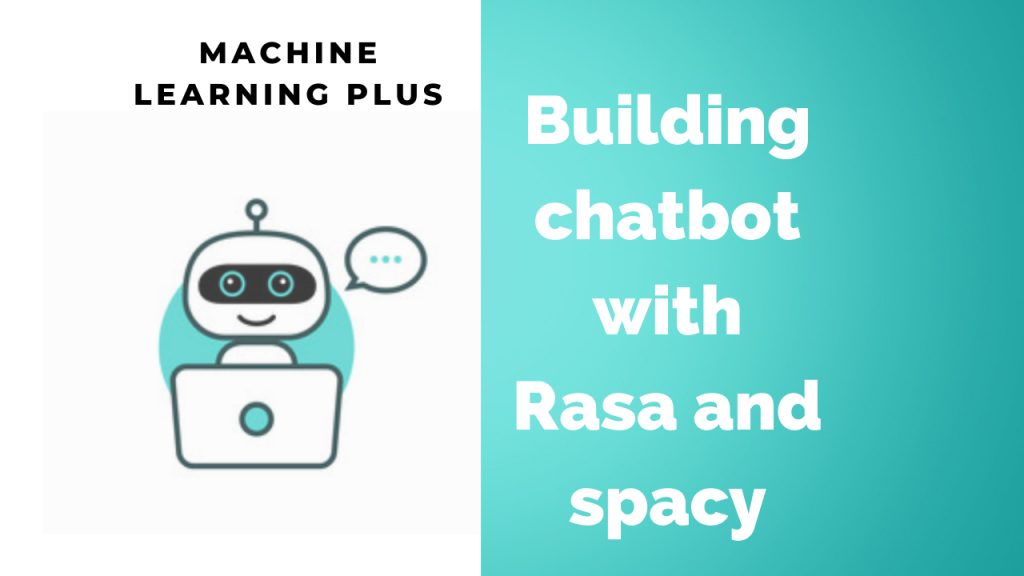## Building chatbot with Rasa and spaCy

Coversational AI systems have revolutionized over the decade. Almost every company faces the requirement to use a ChatBot. Rasa provides a smooth and competitive way to build your own Chatbot. This article will guide you on how to develop your Bot step-by-step simultaneously explaining the concept behind it. Introduction I’m sure each of us would …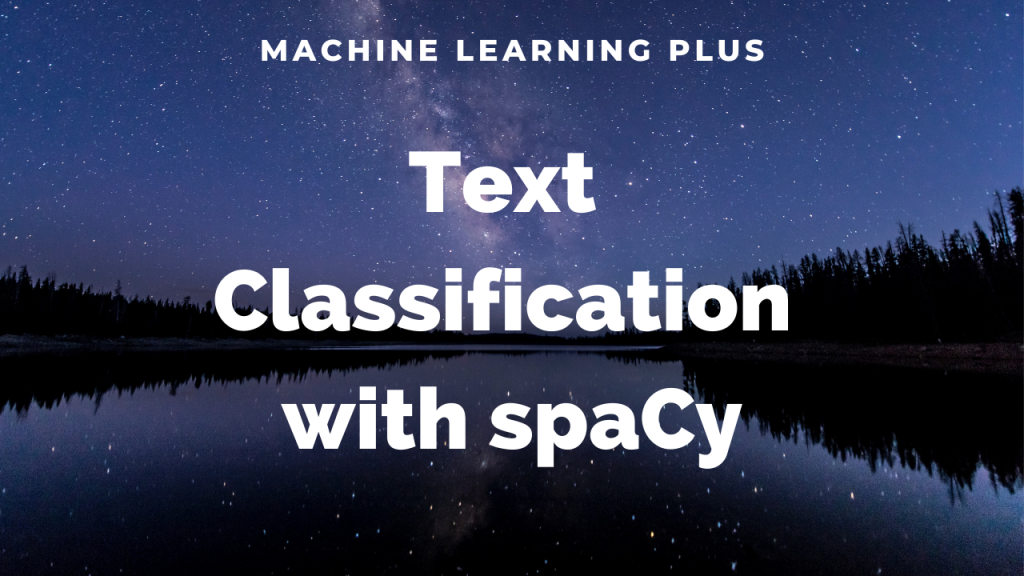## How to Train Text Classification Model in spaCy?

Text Classification is the process categorizing texts into different groups. SpaCy makes custom text classification structured and convenient through the textcat component. Text classification is often used in situations like segregating movie reviews, hotel reviews, news data, primary topic of the text, classifying customer support emails based on complaint type etc. For many real-life cases, …

## How to use tf.function to speed up Python code in Tensorflow

tf.function is a decorator function provided by Tensorflow 2.0 that converts regular python code to a callable Tensorflow graph function, which is usually more performant and python independent. It is used to create portable Tensorflow models.     Spacy for NLP course: Master industry level Natural Language Processing using Spacy. Learn how to setup Spacy, …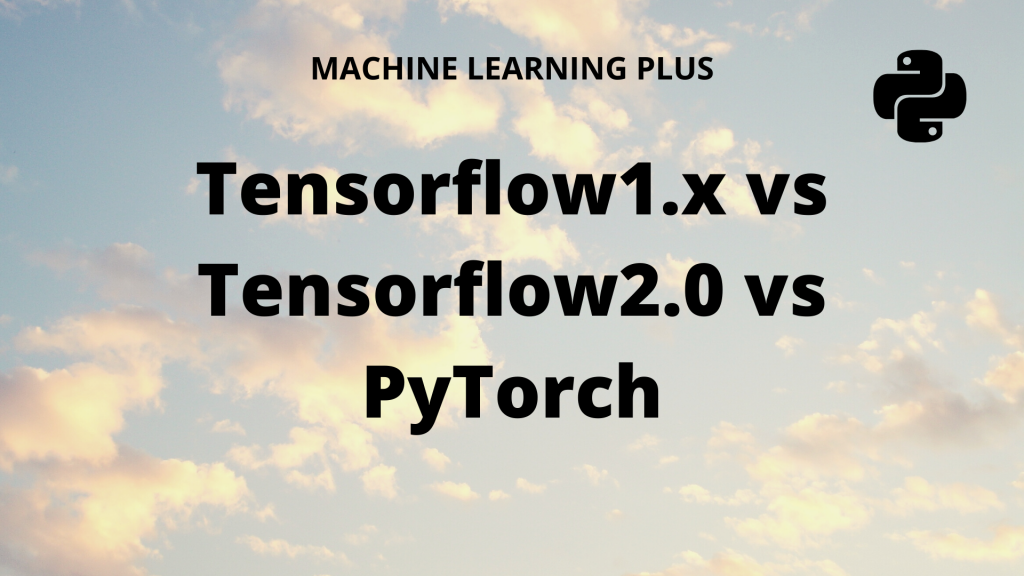## TensorFlow vs PyTorch – A Detailed Comparison

Compare the popular deep learning frameworks: Tensorflow vs Pytorch. We will go into the details behind how TensorFlow 1.x, TensorFlow 2.0 and PyTorch compare against eachother. And how does keras fit in here. Table of Contents: Introduction Tensorflow: 1.x vs 2 Difference between static and dynamic computation graph Keras integration or rather centralization What is …## Linear Regression in Julia

Linear Regression is a fundamental machine learning algorithm used to predict a numeric dependent variable based on one or more independent variables. The dependent variable (Y) should be continuous. In this tutorial I explain how to build linear regression in Julia, with full-fledged post model-building diagnostics. To know more about the concepts behind linear regression, …## For-Loop in Julia

For-loop is a type of loop, that iterates over an iterable object or simply a range of values. It executes some user-defined logic in each iteration. Content Introduction to For-loop in Julia Nested Loop List comprehension in Julia Break Statement in For-loop Continue Statement in For-loop Practice Exercise     Get FREE 30 DAY ACCESS  …## DataFrames in Julia

DataFrame is a 2 dimensional mutable data structure, that is used for handling tabular data. Unlike Arrays and Matrices, a DataFrame can hold columns of different data types The DataFrames package in Julia provides the DataFrame object which is used to hold and manipulate tabular data in a flexible and convenient way. It is quite …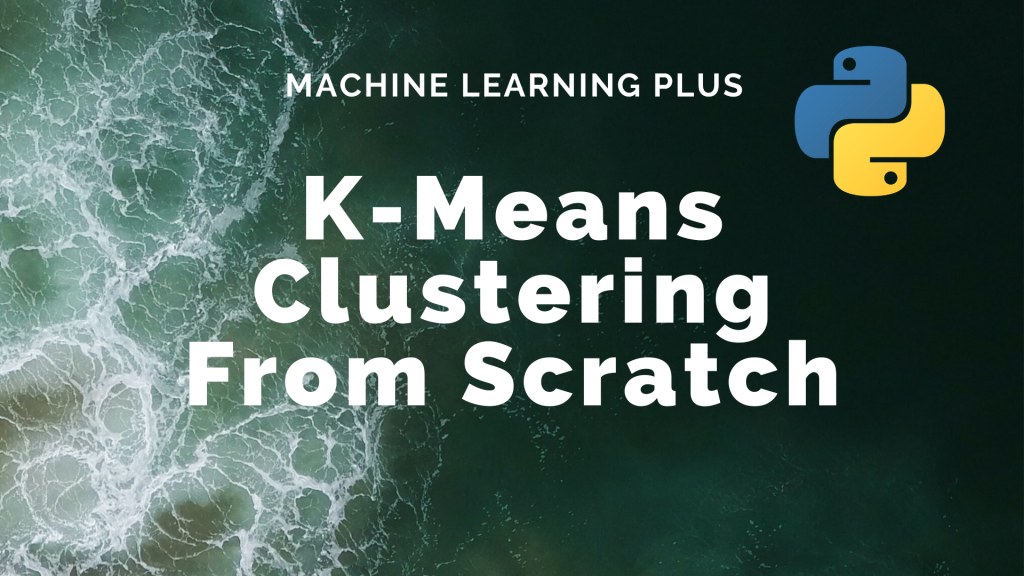## K-Means Clustering Algorithm from Scratch

K-Means Clustering is an unsupervised learning algorithm that aims to group the observations in a given dataset into clusters. The number of clusters is provided as an input. It forms the clusters by minimizing the sum of the distance of points from their respective cluster centroids. Contents Basic Overview Introduction to K-Means Clustering Steps Involved …

## Julia – Programming Language

Julia is a high performance, high-level programming language. It is very popular because of its high speed, machine learning packages and its expressive syntax. It combines the good parts of Python, R, Ruby, Matlab, and Perl and it runs nearly as fast as C. Besides, it’s super easy to use python and R packages within …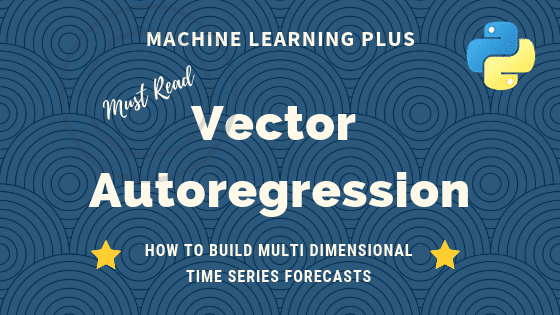## Vector Autoregression (VAR) – Comprehensive Guide with Examples in Python

Vector Autoregression (VAR) is a forecasting algorithm that can be used when two or more time series influence each other. That is, the relationship between the time series involved is bi-directional. In this post, we will see the concepts, intuition behind VAR models and see a comprehensive and correct method to train and forecast VAR …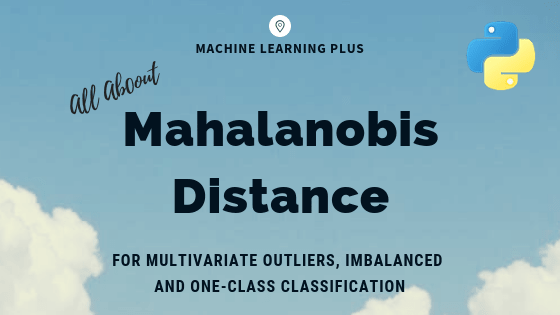## Mahalanobis Distance – Understanding the math with examples (python)

Mahalanobis distance is an effective multivariate distance metric that measures the distance between a point and a distribution. It is an extremely useful metric having, excellent applications in multivariate anomaly detection, classification on highly imbalanced datasets and one-class classification. This post explains the intuition and the math with practical examples on three machine learning use …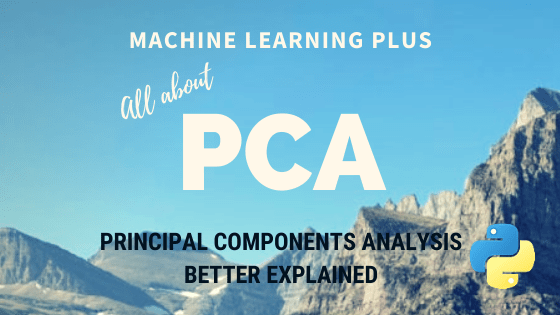## Principal Component Analysis (PCA) – Better Explained

Principal Components Analysis (PCA) is an algorithm to transform the columns of a dataset into a new set of features called Principal Components. By doing this, a large chunk of the information across the full dataset is effectively compressed in fewer feature columns. This enables dimensionality reduction and ability to visualize the separation of classes …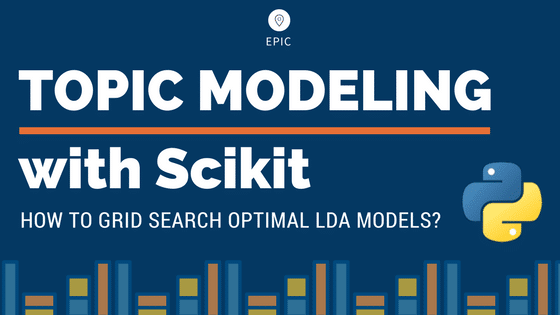## LDA in Python – How to grid search best topic models?

Python’s Scikit Learn provides a convenient interface for topic modeling using algorithms like Latent Dirichlet allocation(LDA), LSI and Non-Negative Matrix Factorization. In this tutorial, you will learn how to build the best possible LDA topic model and explore how to showcase the outputs as meaningful results. Contents 1. Introduction2. Load the packages3. Import Newsgroups Text …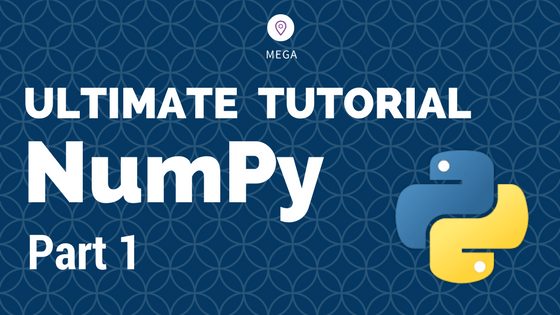## Python Numpy – Introduction to ndarray [Part 1]

This is part 1 of the numpy tutorial covering all the core aspects of performing data manipulation and analysis with numpy’s ndarrays. Numpy is the most basic and a powerful package for scientific computing and data manipulation in python. Numpy Tutorial Part 1: Introduction to Arrays. Photo by Bryce Canyon. Related Posts Numpy Tutorial – …Course Preview

## Machine Learning A-Z™: Hands-On Python & R In Data Science

### Free Sample Videos:#### Machine Learning A-Z™: Hands-On Python & R In Data Science#### Machine Learning A-Z™: Hands-On Python & R In Data Science#### Machine Learning A-Z™: Hands-On Python & R In Data Science#### Machine Learning A-Z™: Hands-On Python & R In Data Science